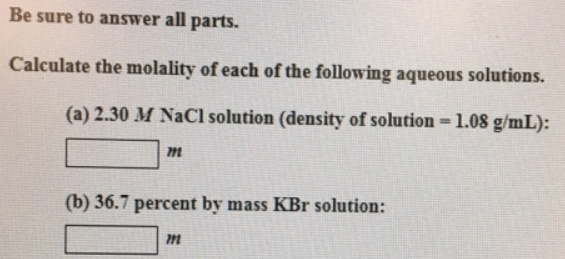# Problem: Be sure to answer all parts.Calculate the molality of each of the following aqueous solutions. (a) 2.30 M NaCl solution (density of solution = 1.08 g/mL): (b) 36.7 percent by mass KBr solution:

###### FREE Expert Solution
79% (369 ratings)###### Problem Details

Be sure to answer all parts.

Calculate the molality of each of the following aqueous solutions.

(a) 2.30 M NaCl solution (density of solution = 1.08 g/mL):

(b) 36.7 percent by mass KBr solution: